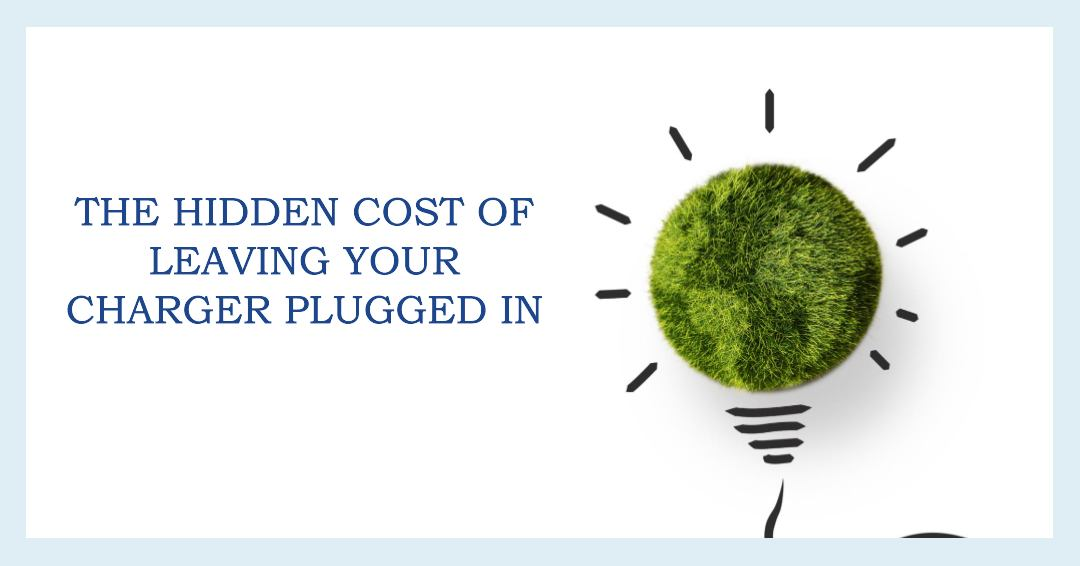# Cost of Keeping Your 5W Plugged In

## Real Cost Breakdown

Electricity usage is calculated in kilowatt-hours. A kilowatt-hour is 1,000 watts used for one hour. As an example, a 100-watt light bulb operating for ten hours would use one kilowatt-hour.To calculate the cost of keeping a 5-watt device charged, you need to consider both the power consumption of the device and the cost of electricity in your area.

Power Consumption: The iPhone Charger consumes 5 watts. This means it consumes 5 watts of electricity per hour of operation.

Cost of Electricity: The cost of electricity varies depending on your location and the pricing structure of your utility company. Electricity is typically billed in kilowatt-hours (kWh). To calculate the cost, you need to know how much you pay per kWh. You can find this information on your electricity bill or by contacting your utility provider.

Once you know the cost per kWh, you can calculate the cost to keep your 5-watt device charged for a specific period of time. Here's the formula:

Cost = (Power Consumption in Watts / 1000) x Hours of Use x Cost per kWh

For example, let's say your electricity costs \$0.12 per kWh, and you want to calculate the cost of keeping your 5-watt device charged for 24 hours:

Cost = (5 watts / 1000) x 24 hours x \$0.12 per kWh Cost = (0.005 kW) x 24 hours x \$0.12 per kWh Cost = 0.12 kWh x \$0.12 per kWh Cost = \$0.0144

So, it would cost you approximately \$0.0144 to keep a 5-watt device charged for 24 hours at a rate of \$0.12 per kWh. Keep in mind that this is a simplified calculation, and the actual cost may vary depending on factors like fluctuations in electricity rates and the efficiency of the charging process.